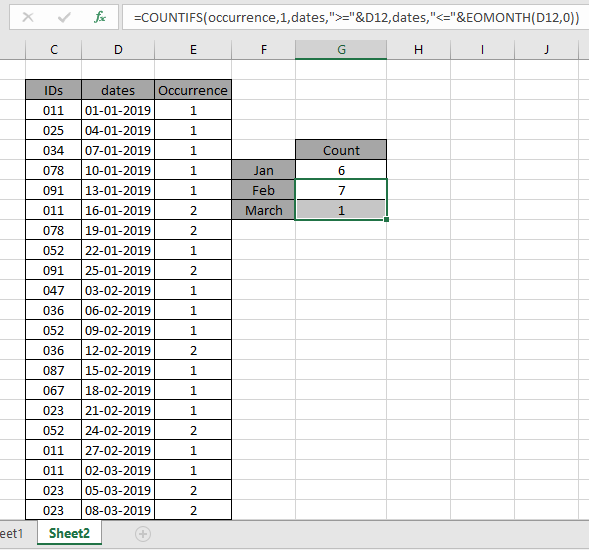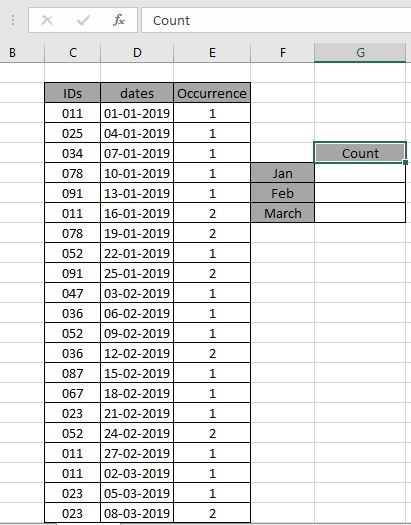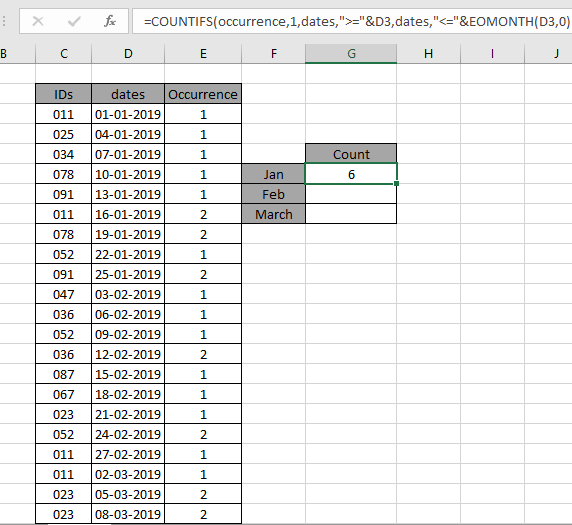# How to Count New Customers Per Month in ExcelIn this article, we will learn how to count new customers per month in excel. In other words, we will need to count only the first appearance of a user in a particular month.

How to solve the problem ?

For this article we will be required to use the COUNTIFS function  and EOMONTH function. Here we are given some values in a range and specific text value as criteria. We need to count the values where the formula includes all the values which ends with the given text or pattern

Generic formula:-

= COUNTIFS ( occurrence, 1, d_rng, ">="& date, d_rng, ">="& EOMONTH( date, 0 )

occurrence: value must match 1.

1: value to match occurrence

d_range : date values as range

date: date value as month criteria

Example:

Here we have the ID records and we need to find unique ID values.I'm here to find the unique values. Some range references using the named range is given.
IDs named range are used for ID values.
dates named range use for date values.
Occurrence named range use for the occurrence count
Use this formula to calculate values in Jan month:

= COUNTIFS ( occurrence , 1 , dates , ">=" & D3 , dates , "<=" & EOMONTH ( D3 , 0 ) )

Explanation:

• COUNTIFS function counts the cells matches occurrence count with.
• Criteria are given as dates greater than or equal to the first date entry of the month.
• & operator concatenate the two values where it lays
• EOMONTH ( D3 , 0 ) criteria is given as dates less than or equal to the last date value of the month.As you can see the total values which occur once in the Jan month comes out to be 6.

Now copy the formula in other cells using the drag down option or using the shortcut key Ctrl + D as shown below. And changing the month criteria to March month and Feb carefully.As you can see all the different values using Excel formulas returns the count of IDs in the data table.

You can also get the count of repeated values in the data table using the below formula.
Use the formula

= COUNTIFS ( occurrence , ">1" , dates , ">=" & D3 , dates , "<=" & EOMONTH ( D3 , 0 ) )

Explanation:

">1" criteria as count matches the occurrence more than one time.

Here are some observational notes shown below.

Notes:

1. The formula only works with numbers and text both
2. Operators like equals to ( = ), less than equal to ( <= ), greater than ( > ) or not equals to ( <> ) can be performed within function applies with number only.

Hope this article about how to Count values which end with using wildcards in Excel is explanatory. Find more articles on COUNTIFS functions here.

If you liked our blogs, share it with your friends on Facebook. And also you can follow us on Twitter and Facebook. We would love to hear from you, do let us know how we can improve, complement or innovate our work and make it better for you. Write us at info@exceltip.com

Related Articles

How to use the SUMPRODUCT function in Excel: Returns the SUM after multiplication of values in multiple arrays in excel.

COUNTIFS with Dynamic Criteria Range : Count cells dependent on other cell values in Excel.

COUNTIFS Two Criteria Match : Count cells matching two different criteria on list in excel.

COUNTIFS With OR For Multiple Criteria : Count cells having multiple criteria match using the OR function.

The COUNTIFS Function in Excel : Count cells dependent on other cell values.

How to Use Countif in VBA in Microsoft Excel : Count cells using Visual Basic for Applications code.

How to use wildcards in excel : Count cells matching phrases using the wildcards in excel

Popular Articles

50 Excel Shortcut to Increase Your Productivity

Edit a dropdown list

Absolute reference in Excel

If with conditional formatting

If with wildcards

Vlookup by date

Terms and Conditions of use

The applications/code on this site are distributed as is and without warranties or liability. In no event shall the owner of the copyrights, or the authors of the applications/code be liable for any loss of profit, any problems or any damage resulting from the use or evaluation of the applications/code.